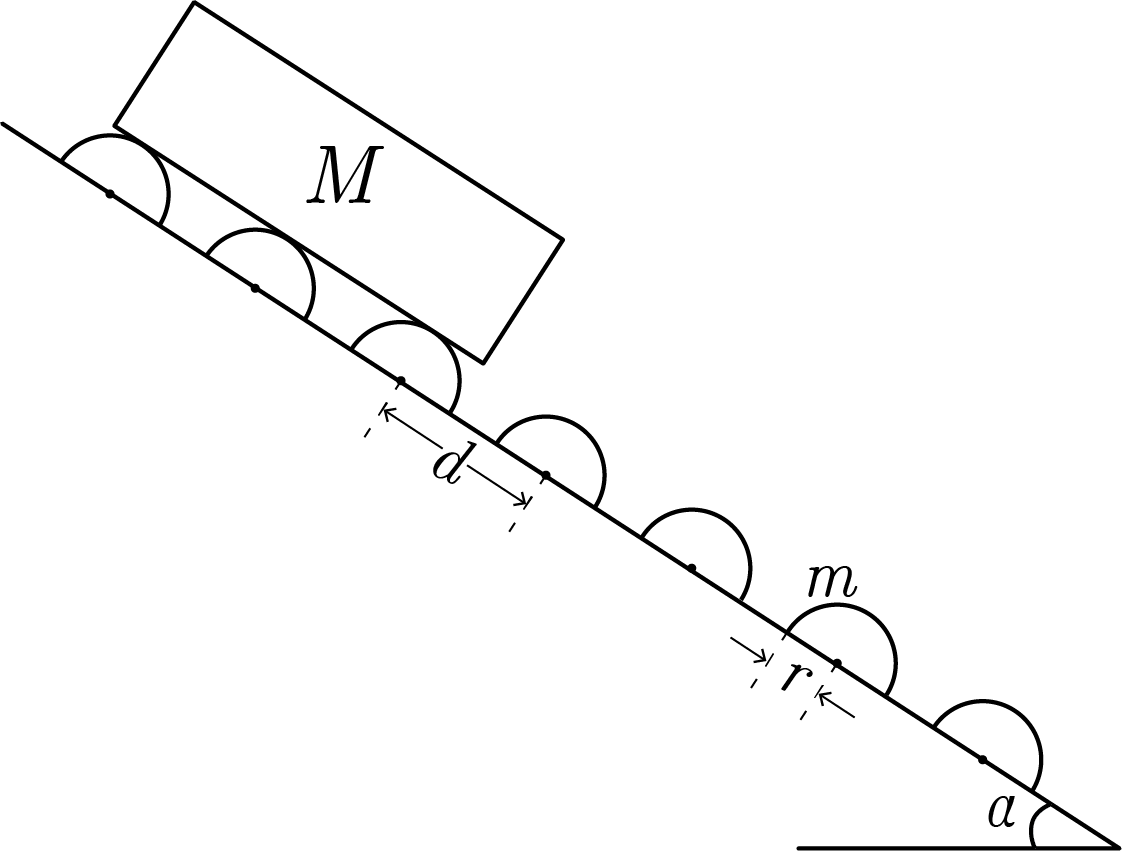# Terminal Trouble

A long slipway is inclined at an angle $\alpha$ to the horizontal and is fitted with a series of identical rollers of mass $m$ and radius $r,$ a distance $d$ apart along the slipway. A plank of length $L\gg d$ and mass $M = 20m$ is released from the top of the slipway, and eventually reaches some terminal velocity $v_\infty.$Find $v_\infty$ and report your answer to the nearest integer.

Assumptions

• When the plank is at terminal velocity, the rollers obtain $\omega_\infty = v_\infty/r$ just before losing contact with the plank.
• $\sin \alpha = \frac12,$ $r = \SI{0.2}{\meter},$ $d=\SI{1}{\meter},$ $g=\SI[per-mode=symbol]{9.81}{\meter\per\second\squared}.$
×